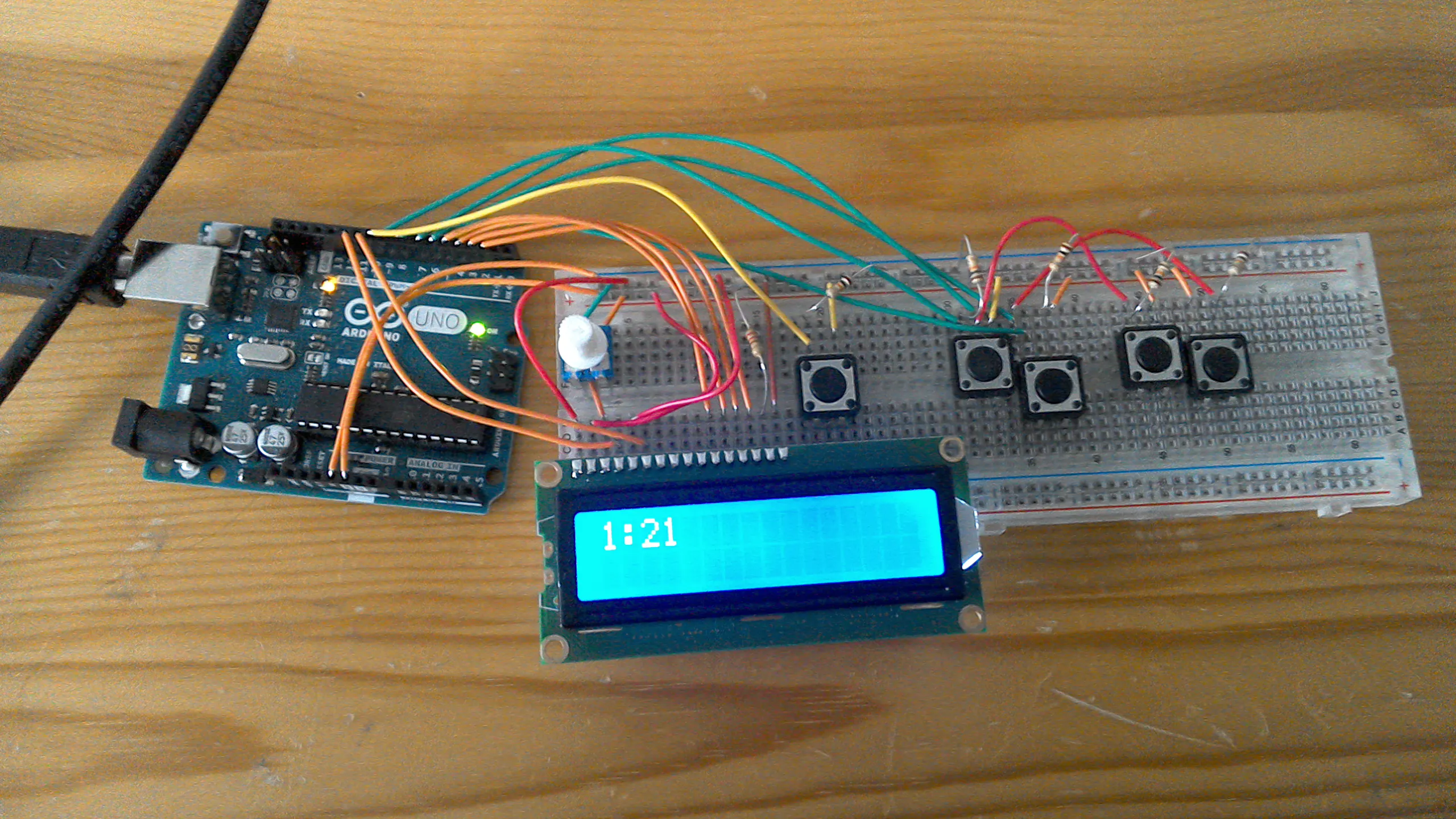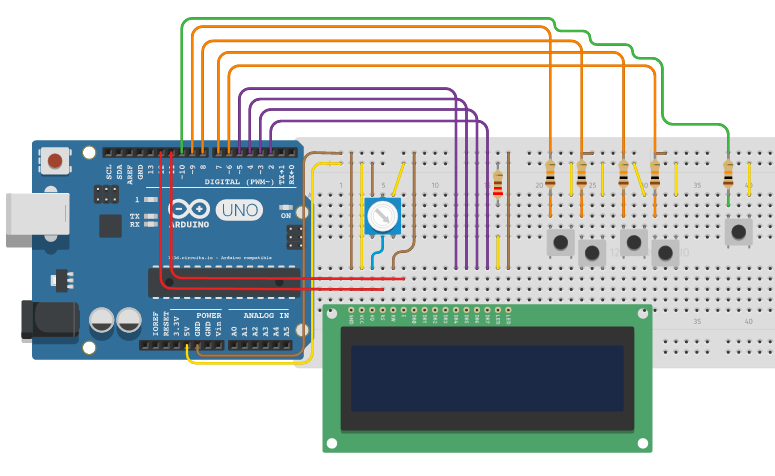Project in progress# Digital Clock © GPL3+

Basically a digital clock that can have the time changed at the press of a button.

• 13,447 views
• 6 comments
• 35 respects

## About this project

My clock works like this, every 60 seconds the minutes go up by 1. every 60 minutes the hours go up by one, and when the hours get to 13, they change to 1. We all know how clocks work. Then if you hold down the change time button for one second, you are able to change the time using the other buttons, as shown in the video.I don't know why I decided to make this, I just thought it would be a fun learning experience. Here is the video of me demonstrating it. Once I eventually get a 3D printer, I will turn this into a fully fledged clock. Sorry about the looks.

## Code

##### My code!C/C++
Here is the code in case anybody wants to try it for themselves.
```#include <LiquidCrystal.h>
//to change time, hold chanage time button for 1 second, then use hour chnage and minute change buttons.

LiquidCrystal lcd(12, 11, 5, 4, 3, 2);
int hour = 12;
int minutes = 0;
int seconds = 0;
int decBtn = 10;
int hrUp = 9;
int hrDwn = 8;
int minUp = 7;
int minDwn = 6;
int hrUpDec = 0;
int hrDwnDec = 0;
int minUpDec = 0;
int minDwnDec = 0;

void setup() {
lcd.begin(16, 2);
pinMode(decBtn, INPUT);
pinMode(hrUp, INPUT);
pinMode(hrDwn, INPUT);
pinMode(minUp, INPUT);
pinMode(minDwn, INPUT);
}

void loop() {
if (digitalRead(decBtn) == HIGH) {
if (digitalRead(hrUp) == HIGH && hrUpDec == 0) {
hrUpDec = 1;
hour = hour + 1;
} else if (digitalRead(hrUp) == LOW) {
hrUpDec = 0;
}
if (digitalRead(hrDwn) == HIGH && hrDwnDec == 0) {
hrDwnDec = 1;
hour = hour - 1;
} else if (digitalRead(hrDwn) == LOW) {
hrDwnDec = 0;
}
if (digitalRead(minUp) == HIGH && minUpDec == 0) {
minUpDec = 1;
minutes = minutes + 1;
} else if (digitalRead(minUp) == LOW) {
minUpDec = 0;
}
if (digitalRead(minDwn) == HIGH && minDwnDec == 0) {
minDwnDec = 1;
minutes = minutes - 1;
} else if (digitalRead(minDwn) == LOW) {
minDwnDec = 0;
}
seconds = 0;
lcd.clear();
if (minutes == 60) {
minutes = 0;
hour = hour + 1;
}
if (minutes < 0) {
minutes = 59;
}
if (hour == 13) {
hour = 1;
}
if (hour < 1) {
hour = 12;
}
lcd.print(hour);
lcd.print(":");
if (minutes < 10) {
lcd.print(0);
}
lcd.print(minutes);
delay(20);
} else if (digitalRead(decBtn) == LOW) {
lcd.clear();
if (seconds == 60) {
seconds = 0;
minutes = minutes + 1;
}
if (minutes == 60) {
minutes = 0;
hour = hour + 1;
}
if (minutes < 0) {
minutes = 59;
}
if (hour == 13) {
hour = 1;
}
if (hour < 1) {
hour = 12;
}
lcd.print(hour);
lcd.print(":");
if (minutes < 10) {
lcd.print(0);
}
lcd.print(minutes);
delay(1000);
lcd.clear();
seconds = seconds + 1;
}
}
```

## Schematics

Just the schematic.## Comments

#### Simple Arduino Digital Clock Without RTC

Project in progress by Annlee Fores

• 123,960 views
• 32 comments
• 111 respects

#### Clock Set Date Time

Project tutorial by Tittiamo

• 59,178 views
• 52 comments
• 75 respects

#### Ternary Digital Clock with Arduino

Project tutorial by LAGSILVA

• 6,003 views
• 6 comments
• 15 respects

#### Complete Digital Clock Including Alarm and Motion Sensor

Project tutorial by LAGSILVA

• 21,314 views
• 22 comments
• 73 respects

#### Tri-Mode Digital Clock with ATtiny85 and RTC

Project tutorial by LAGSILVA

• 8,833 views
• 4 comments
• 25 respects

#### Digital Clock with Arduino, RTC and Shift Register 74HC595

Project tutorial by LAGSILVA

• 33,226 views
• 26 comments
• 75 respects